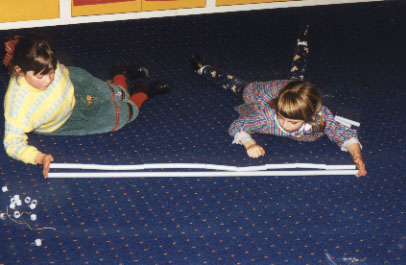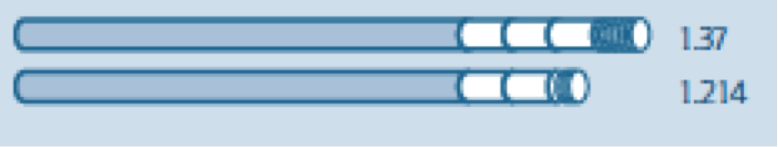Introducing and comparing decimal numbers can sometimes be a confusing concept for students, who still associate visually larger numbers as mathematically larger numbers. Students must be able to understand and work fluently with counting collections to 100 by grouping in tens, and counting the tens, and use place value to partition and regroup those numbers.  When beginning their study of place value, students correctly state that 1789 is larger than 892 because it has more digits. However, the same is not true of decimal numbers, where 8.742 is larger than 8.1576.

Consider the following extract taken from the Department of Education and Early Childhood Development:

Success depends on students realising that it is the digits with the highest place value that contribute the most to the size of a number. This is especially difficult for decimal numbers.   Young students often think that the size of a decimal number is related to the length of the decimal, because they draw false analogies with whole numbers. For example, they might think that ‘1576 is larger than 742 as it is contains more digits’ and so conclude that ‘8.1576 is bigger than 8.742 as it is longer’. Understanding decimal numbers requires a deeper understanding of place value.

Linear Arithmetic Blocks (LABs) are thus an excellent tool for teaching young students about the components of decimal numbers. They provide a visual for ones, tenths, hundredths and thousandths.   Students can use LABs as a visual to-scale model of decimal place value. The numbers are represented by increasingly smaller lengths of piping, with the largest pipe representing “one,” and the smallest representing a “thousandth.”   Students are shown the largest section of pipe, depicting “one”, first. They are then asked to discuss cutting it into ten equal pieces, or “tenths”.   The “tenth” piece of pipe is then shown to students, and students are asked to discuss cutting it into ten equal pieces. They are asked to predict the size of the subsequent pipe and to give a name for it – “hundredths.”   This continues until students are left with “thousandths”.Students use their LAB pipes in the classroom. Image via The University of Melbourne extranet

The use of LABs is ideal as it uses the physical quantity of length to represent the size of a number. Students are thus able to see that digits with the highest place value contributes the most to the size of a number. For instance, the below visualisation of a LAB depicts the numbers 1.37 and 1.214. It is now clear to students that despite 1.214 having more digits, 1.37 is still the larger number.Download AMSI’s free Place Value presentation for more ideas and information on teaching place value, numeration, decimals and counting.

For additional information on comparing decimal numbers from the Department of Education and Early Childhood Development, click here. The Department also has a post focusing specifically on LAB, which can be found here.

Recent Posts